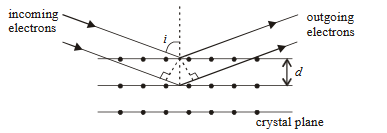Q

# I have a doubt, kindly clarify. - Dual Nature of Matter and Radiation - JEE Main

Question is based on the following paragraph.

Wave property of electrons implies that they will show diffraction effects. Davisson and Germer demonstrated this by diffracting electrons from crystals. The law governing the diffraction from a crystal is obtained by requiring that electron waves reflected from the planes of atoms in a crystal interfere constructively (see figure).Question : If a strong diffraction peak is observed when electrons are incident at an angle from the normal to the crystal planes with distance between them (see figure), de Broglie wavelength of electrons can be calculated by the relationship ( is an integer )

 Option 1) Option 2) Option 3) Option 4)
127 Views

As we learnt in

Bragg’s formula -

- wherein

From condition of constructive interference of Bragg's law:

Option 1)

This is an incorrect option.

Option 2)

This is an incorrect option.

Option 3)

This is the correct option.

Option 4)

This is an incorrect option.

Exams
Articles
Questions